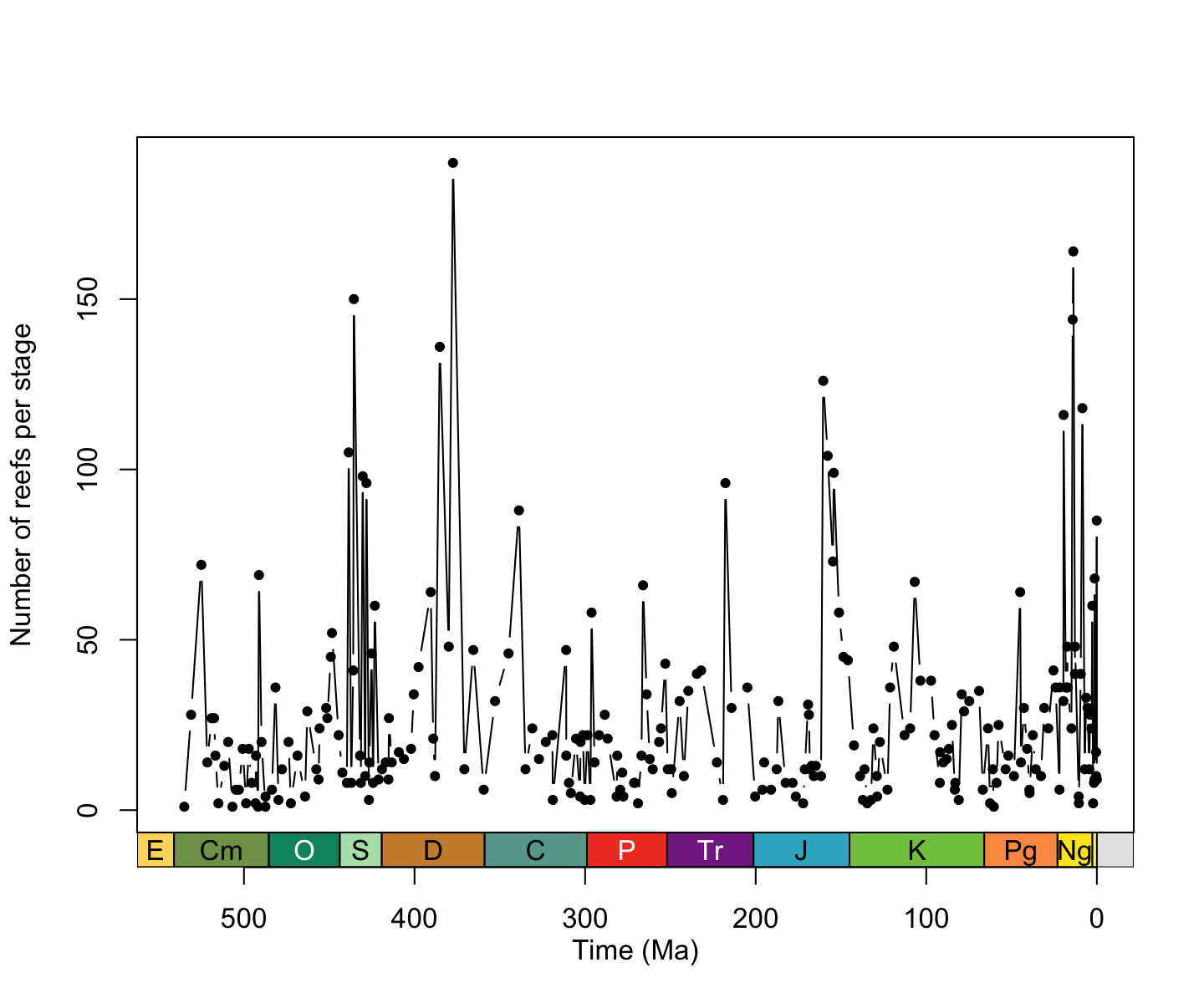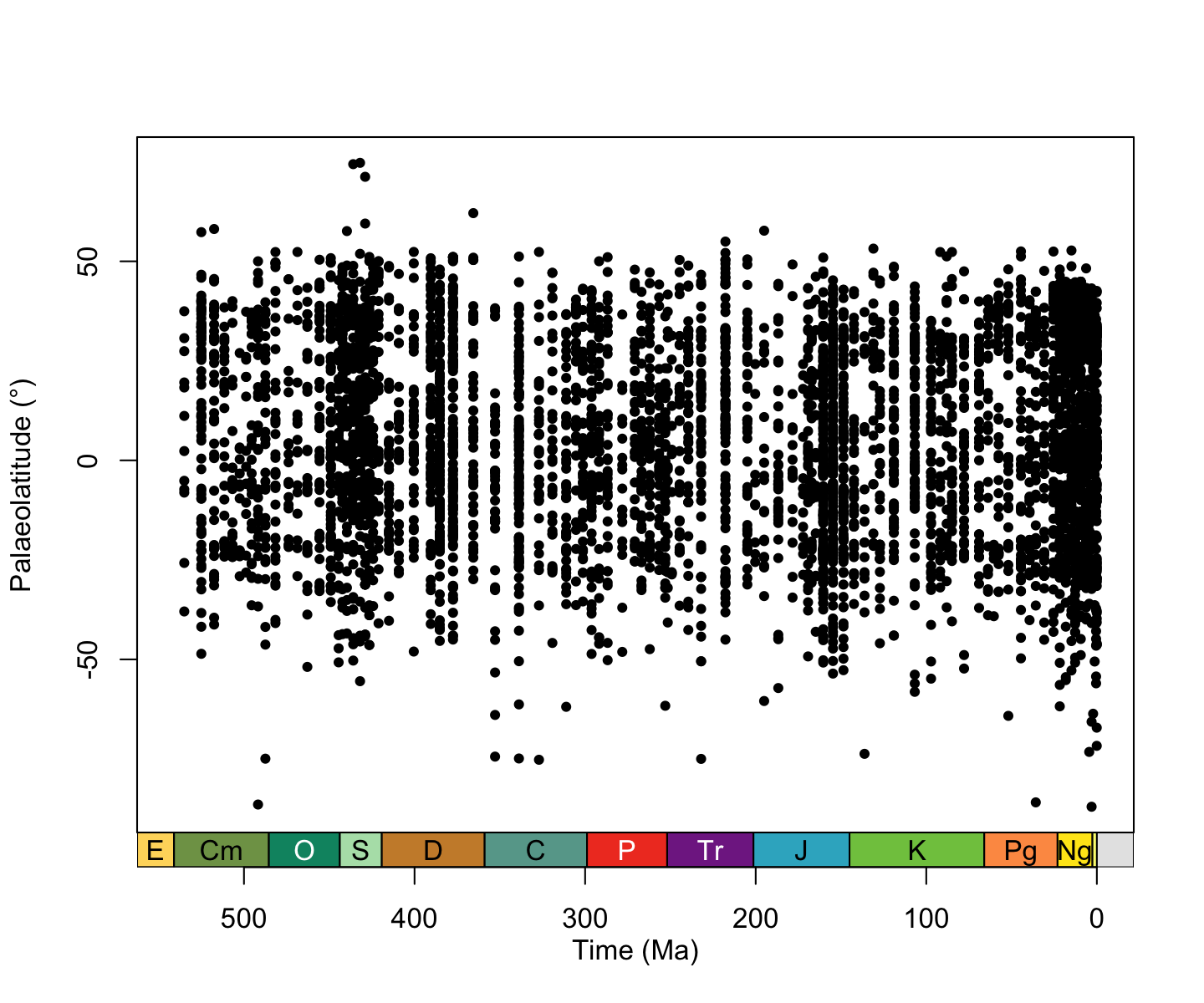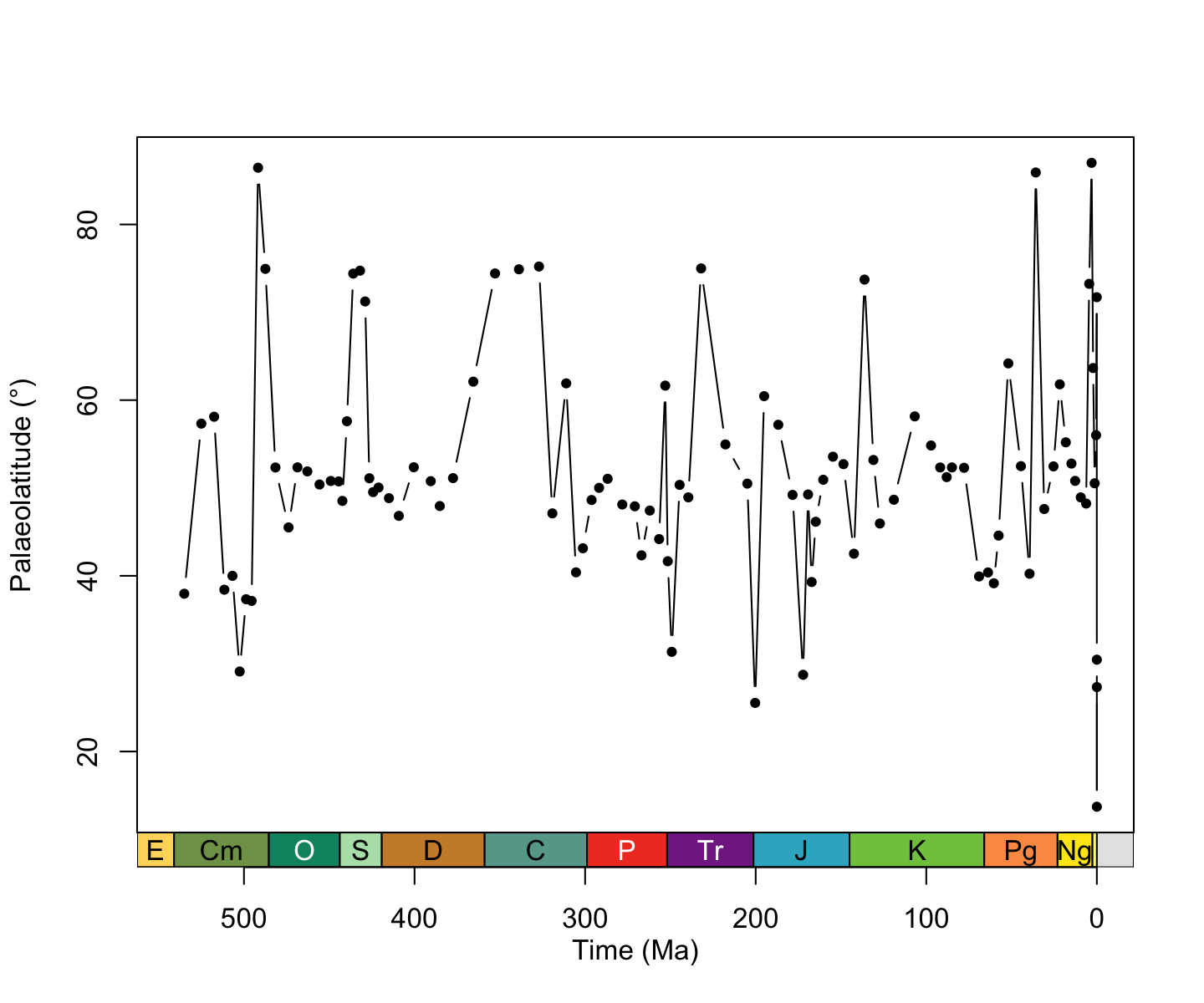# Latitudinal trends in Phanerozoic reefs

Authors: The Palaeoverse Development Team

Last updated: 2023-04-18

# Introduction

`palaeoverse` is an R package developed by palaeobiologists, for palaeobiologists. The aim of the package is to generate a community-driven toolkit of generic functions for the palaeobiological community. The package does not provide implementations of statistical analyses, rather it provides auxiliary functions to help streamline analyses and improve code readability and reproducibility. This vignette (or tutorial if you prefer) is provided to guide you through the installation process and some of the functionality available within `palaeoverse`. To do so, we will focus on a usage example looking at latitudinal trends in Phanerozoic reefs.

## Installation

The `palaeoverse` package can be installed via CRAN or its dedicated GitHub repository if the development version is preferred. To install via the CRAN, simply use:

``install.packages("palaeoverse")``

To install the development version, first install the `devtools` package, and then use `install_github` to install `palaeoverse` directly from GitHub.

``````install.packages("devtools")
devtools::install_github("palaeoverse-community/palaeoverse")``````

You can now load `palaeoverse` using the default `library` function:

``library(palaeoverse)``

Before we get onto the good stuff, the development team has a small request. If you use `palaeoverse` in your research, please cite the associated publication. This will help us to continue our work in supporting you to do yours. You can access the appropriate citation via:

``citation("palaeoverse")``

## Using the package

Functions in `palaeoverse` are designed around the assumption that most users’ data are stored in an occurrence `data.frame`. As such, this is the expected input data format for most functions. We provide two example occurrence datasets (`tetrapods` and `reefs`) within the package from different data sources. In this vignette, we will focus on the `reefs` dataset which is a compilation of Phanerozoic reef occurrences (n = 4,363) from the PaleoReefs Database (Kiessling & Krause, 2022).

``````# Load the dataset
data(reefs)
# View the first five rows & columns of reefs
reefs[1:5, 1:5]``````
``````##   r_number               name             formation   system         series
## 1        1   Tilkideligi Tepe                       Triassic Upper Triassic
## 2        2              Hydra Pantokrator Limestone Triassic Upper Triassic
## 3        3 Shiraho, W-Pacific                        Neogene       Holocene
## 4        4 Aksu-Terziler area                       Triassic Upper Triassic
## 5        5    Adnet, Salzburg                       Triassic Upper Triassic``````

Note the structure of the dataset. Each row contains a unique occurrence and each column contains a unique variable.

# Let’s begin!

Reefs have long been considered good tracers of past tropical and subtropical conditions (Kiessling, 2001; Perrin & Kiessling, 2010). More specifically, their distribution has historically been used to infer the latitudinal extent of tropical and subtropical climatic conditions in deep time. This is of course an oversimplification given all we know about modern reefs systems and their engineers. However, for the purpose of this example, let’s not get weighed down by the finer details.

In this example, we will use the `reefs` dataset to examine latitudinal trends in Phanerozoic reefs and demonstrate some of the functionality available in `palaeoverse`.

Let’s start by exploring the dataset. Before conducting any analyses, it is always a good idea to explore your data and understand what you are working with. Details related to the example datasets can be accessed via the usual documentation call:

``````# Call documentation for dataset
?reefs
# You can also use
help(reefs)``````

Let’s start by exploring the data a little:

``````# How many reefs are there in the dataset?
# Each row represents an individual reef.
nrow(reefs)``````
``##  4363``

Not bad (n = 4363), that’s quite a bit of data to play with! Remember that these are reefs (whole ecosystems), not individual occurrences of fossil organisms like in `tetrapods`. But how much does this vary across time? We can make use of the `group_apply` wrapper function to run a function across subsets of data.

``````# How many reefs per interval?
# Let's group by the interval column to test
reef_counts <- group_apply(occdf = reefs, group = "interval", fun = nrow)
``````##   nrow                interval
## 1    2       (early?) Toarcian
## 2    1       Aalenian-Bajocian
## 3    4       Aalenian/Bajocian
## 4    1 Aalenian/lower Bajocian
## 6    6                Aeronian``````

Oh dear, we’ve just hit a common issue… our reef data does not conform to a common time scale! Some reefs are resolved to stage level, while others are resolved to a coarser or finer temporal resolution. In some instances, regional names are also used, while international names are used in others. This makes it challenging to make comparisons through time… one solution could be to use age values to bin the reefs into time bins, but no age range data is provided in the dataset (i.e. “max_ma” and “min_ma”). We must therefore use the names of each interval to link to a common time scale, the ‘International Geological Stages’ established by the International Commission on Stratigraphy (ICS). Fortunately, we can make use of the `look_up` function to do so. This function can be used to assign international geological stages and numeric ages to occurrences, or use a user-defined interval key to link to a common time scale. Here, we will use the example `interval_key` available in `palaeoverse` to assign international geological stage names and numeric ages.

``````# Load the interval key
data("interval_key")
# Assign a common time scale based on an interval key
reefs <- look_up(occdf = reefs,
early_interval = "interval",
late_interval = "interval",
int_key = interval_key)
# Example output
reefs[100:110, 15:19]``````
``````##     early_stage late_stage interval_max_ma interval_mid_ma interval_min_ma
## 100    Ladinian    Carnian           242.0          234.50           227.0
## 101      Norian     Norian           227.0          217.75           208.5
## 102    Rhaetian   Rhaetian           208.5          204.90           201.3
## 103    Ladinian    Carnian           242.0          234.50           227.0
## 104      Norian   Rhaetian           227.0          214.15           201.3
## 105     Anisian    Anisian           247.2          244.60           242.0
## 106    Rhaetian   Rhaetian           208.5          204.90           201.3
## 107  Sinemurian Sinemurian           199.3          195.05           190.8
## 108   Oxfordian  Oxfordian           163.5          160.40           157.3
## 109   Tithonian  Tithonian           152.1          148.55           145.0
## 110   Tithonian  Tithonian           152.1          148.55           145.0``````

Great! We now have a common time scale and numeric ages. However, some of our reefs seem to range through several stages and we can’t count just by the early or late stage. Our data still need to be binned. Let’s check out the `time_bins` and `bin_time` functions.

``````# Now we have numeric ages for our data, we can easily
# remove pre-Phanerozoic data to focus our study
reefs <- subset(reefs, interval_max_ma <= 541)
# Extract Phanerozoic stage-level stages for time bins
bins <- time_bins(interval = "Phanerozoic", rank = "stage")
# Bin data
# bin_time requires "max_ma" and "min_ma" columns
# Rename columns in reefs
colnames(reefs)[which(colnames(reefs) == "interval_max_ma")] <- "max_ma"
colnames(reefs)[which(colnames(reefs) == "interval_min_ma")] <- "min_ma"``````
``````# Five methods exist in bin_time for binning occurrence data
# You can see details on each via ?bin_time
# Bin by midpoint age
bin_time(occdf = reefs, bins = bins, method = "mid")
# Bin by overlap majority
bin_time(occdf = reefs, bins = bins, method = "majority")
# Bin into every bin within age range
bin_time(occdf = reefs, bins = bins, method = "all")
# Bin randomly into bins within age range (equal probability)
bin_time(occdf = reefs, bins = bins, method = "random", reps = 10)
# Bin randomly into bins within age range (uniform probability)
bin_time(occdf = reefs, bins = bins, method = "point", reps = 10)``````
``````# Let's go with "all" for this example!
reefs <- bin_time(occdf = reefs, bins = bins, method = "all")``````

Note that the number of rows of `reefs` have now increased. This is because the “all” method in `bin_time` duplicates an occurrence for every bin the age range overlaps with.

Let’s check those reef numbers through time again using our binned data:

``````# Count number of occurrences per interval
reefs_time <- group_apply(occdf = reefs, group = "interval_mid_ma", fun = nrow)
# Check output
``````##   nrow interval_mid_ma
## 1    9         0.00585
## 2   85         0.07035
## 3   10         0.39285
## 4   17          0.4515
## 5   68         1.29585
## 6    9          1.3545``````

That’s more like it! But, let’s not forget it’s always useful to visualise our data. Why don’t we plot the number of reefs through time? We can even make use of the `axis_geo` function to add the Geological Time Scale to our plot.

``````# Plot data
plot(x = reefs_time\$interval_mid_ma,
y = reefs_time\$nrow,
xlab = "Time (Ma)",
ylab = "Number of reefs per stage",
xlim = c(541, 0),
xaxt = "n",
type = "b", pch = 20)
# Add axis of the geological time scale
axis_geo(side = 1, intervals = "periods")``````You would (and should) of course want to explore your data a little more than this. However, for the sake of brevity, let’s focus on our research theme: latitudinal trends in Phanerozoic reefs.

When studying modern latitudinal trends (e.g. in biodiversity), researchers can use the geographic coordinates of their samples to conduct analyses. However, as the continents shift through time, palaeobiologists must reconstruct the palaeogeographic coordinates of fossil localities. To do so, plate rotation models are used (e.g. the PALEOMAP model) to rotate the modern coordinates of fossil localities to their location at time of deposition. The `palaeorotate` function allows the user to do so using either the ‘point’ or ‘grid’ method. The first approach makes use of the GPlates Web Service and allows point data to be rotated to specific ages using the available models (see https://gwsdoc.gplates.org). The second approach uses reconstruction files of pre-generated palaeocoordinates to spatiotemporally link occurrences’ modern coordinates and age estimates with their respective palaeocoordinates. Let’s give it a shot:

``````# Palaeorotate occurrences
reefs <- palaeorotate(occdf = reefs, age = "bin_midpoint",
method = "point", model = "PALEOMAP")``````
``````# Check palaeocoordinates
``````##      p_lng   p_lat
## 1  21.3630 10.0755
## 2  17.4435 25.5719
## 3 -72.6726 27.3965
## 4  17.9163 13.7121
## 5  18.0122 25.2688
## 6  16.5789 24.6727``````

Now let’s plot that palaeolatitudinal distribution!

``````# Plot data
plot(x = reefs\$bin_midpoint,
y = reefs\$p_lat,
xlab = "Time (Ma)",
ylab = "Palaeolatitude (\u00B0)",
xlim = c(541, 0),
xaxt = "n",
type = "p", pch = 20)
axis_geo(side = 1, intervals = "periods")``````Neat! But it’s hard to draw any trends from this data (there is a lot of it!). We can do better… let’s summarise this information… if we assume that reefs are broadly limited to tropical and subtropical climatic conditions, the most poleward reef in each time bin should give us an estimate of the palaeolatitudinal extent of these climatic conditions.

``````# Let's first assume hemispheric symmetry and convert
# palaeolatitudes to absolute palaeolatitudes
reefs\$p_lat <- abs(reefs\$p_lat)
# Now we can calculate the most poleward latitude per time bin
# Extract unique interval midpoints
midpoints <- sort(unique(reefs\$bin_midpoint))
# Calculate the maximum palaeolatitude for each time bin
reefs_max <- sapply(X = midpoints, FUN = function(x) {
max(reefs[which(reefs\$bin_midpoint == x), ]\$p_lat, na.rm = TRUE)
} )
# Plot data
plot(x = midpoints,
y = reefs_max,
xlab = "Time (Ma)",
ylab = "Palaeolatitude (\u00B0)",
xlim = c(541, 0),
xaxt = "n",
type = "b",
pch = 20)
# Add axis of the geological time scale
axis_geo(side = 1, intervals = "periods")``````That’s definitely much clearer! Should we stop there? Well… how about one last thing… let’s consider how plate rotation model choice might impact these results using three different models: GOLONKA, PALEOMAP, and MERDITH2021 (Wright et al. 2013; Scotese & Wright, 2018; Merdith et al. 2021).

``````# We can call multiple models at once with palaeorotate
# First, let's define the models...
models <- c("GOLONKA", "PALEOMAP", "MERDITH2021")
# And now palaeorotate!
reefs <- palaeorotate(occdf = reefs, age = "bin_midpoint",
method = "point", model = models)``````
``````# Check palaeocoordinates
# When multiple models are called, the name of the model
# is added as a suffix to p_lng and p_lat
"p_lng_GOLONKA", "p_lat_GOLONKA",
"p_lng_MERDITH2021", "p_lat_MERDITH2021")])``````
``````##   p_lng_PALEOMAP p_lat_PALEOMAP p_lng_GOLONKA p_lat_GOLONKA p_lng_MERDITH2021
## 1        21.3630        10.0755       35.6565        8.3384           34.4230
## 2        17.4435        25.5719       32.2609       19.9044           21.1582
## 3       -72.6726        27.3965      -47.2123       34.6578          -46.7249
## 4        17.9163        13.7121       29.2657        8.3957           29.2360
## 5        18.0122        25.2688       32.6906       19.4663           21.8226
## 6        16.5789        24.6727       31.1897       19.2698           27.9955
##   p_lat_MERDITH2021
## 1           12.1941
## 2           26.5767
## 3           37.2704
## 4           12.8023
## 5           26.5278
## 6           21.6152``````

Now we’ve palaeorotated our data, let’s repeat what we did earlier and calculate the most poleward reef occurrence for each model.

``````# Let's code a little helper function to begin with.
# This is generally useful when repeating code several times!
p_lat_max <- function(occdf, midpoint, p_lat) {
# Get absolute palaeolatitudes
occdf[, p_lat] <- abs(occdf[, p_lat])
# Extract unique bin midpoints
midpoints <- sort(unique(occdf[, midpoint]))
# Calculate maximum palaeolatitude for each bin
lat_max <- sapply(X = midpoints, FUN = function(x) {
max(occdf[which(occdf[, midpoint] == x), ][, p_lat], na.rm = TRUE)
})
# Bind data
lat_max <- cbind.data.frame(midpoints, lat_max)
# Return df
return(lat_max)
}
# Calculate maximum palaeolatitude of reefs for each time bin for each model
paleomap <- p_lat_max(occdf = reefs,
midpoint = "bin_midpoint",
p_lat = "p_lat_PALEOMAP")
golonka <- p_lat_max(occdf = reefs,
midpoint = "bin_midpoint",
p_lat = "p_lat_GOLONKA")
merdith <- p_lat_max(occdf = reefs,
midpoint = "bin_midpoint",
p_lat = "p_lat_MERDITH2021")
# Set up plot
plot(x = NULL,
y = NULL,
xlab = "Time (Ma)",
ylab = "Palaeolatitude (\u00B0)",
xlim = c(541, 0),
ylim = c(0, 90),
xaxt = "n")
# Plot maximum palaeolatitudes for each model
lines(x = paleomap\$midpoints, y = paleomap\$lat_max,
type = "o", col = "#e41a1c", pch = 15)
lines(x = golonka\$midpoints, y = golonka\$lat_max,
type = "o", col = "#377eb8", pch = 16)
lines(x = merdith\$midpoints, y = merdith\$lat_max,
type = "o", col = "#4daf4a", pch = 17)